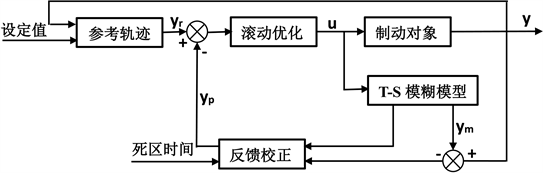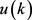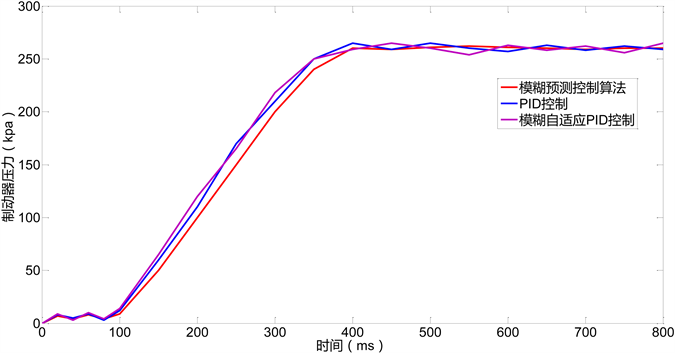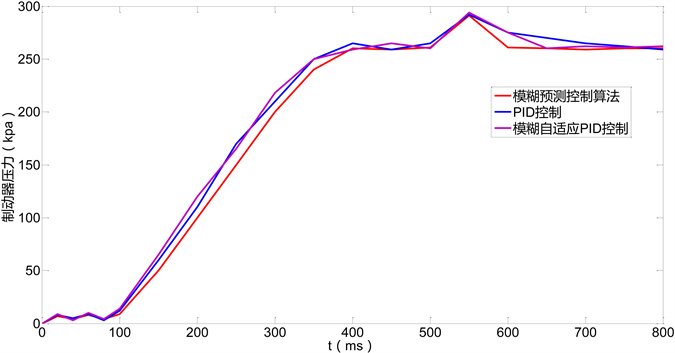﻿ 基于T-S模糊模型的预测控制算法在城轨列车制动控制中的应用

# 基于T-S模糊模型的预测控制算法在城轨列车制动控制中的应用Application of Predictive Control Algorithm Based on T-S Fuzzy Model in Urban Rail Train Braking Control

Abstract: Urban rail transit plays an increasingly important role in modern urban life because of its large carrying capacity, fast speed and zero blockage. Braking system performance directly affects the smoothness and safety of train operation, which is a time-varying, time-delay and nonlinear control system. A predictive control algorithm based on T-S fuzzy model is proposed. The simulation of urban rail train braking control system can realize fast and accurate synchronous braking, overcome the dynamics of uncertainties, hysteresis and time-varying of controlled objects. So it can achieve the desired control objectives and make the system have robust and stable performance.

1. 引言

2. 基于T-S模糊模型的广义预测控制算法

2.1. T-S模糊模型Figure 1. Structure diagram of train predictive control system based on T-S fuzzy model

${R}_{i}:\text{If}{x}_{1}\text{is}\text{\hspace{0.17em}}{f}_{1}^{t}\text{and}{x}_{2}\text{is}\text{\hspace{0.17em}}{f}_{2}^{t}\text{and}\cdots \text{and}{x}_{m}\text{is}\text{\hspace{0.17em}}{f}_{m}^{t}$ (1)

$\text{Then}:\stackrel{˙}{x}={A}_{i}x+{B}_{i}u$ (2)

$\stackrel{˙}{x}=\underset{i=1}{\overset{l}{\sum }}{\omega }_{i}\left({A}_{i}x+{B}_{i}u\right)/\underset{i=1}{\overset{l}{\sum }}{\omega }_{i}$ (3)

$\stackrel{˙}{x}=\underset{i=1}{\overset{l}{\sum }}{h}_{i}\left({A}_{i}x+{B}_{i}u\right)$ (4)

T-S模糊模型的建立主要分为结构辨识和参数辨识。与结构辨识相比，模糊模型的参数辨识要相对容易和成熟的多，主要可以分为两个大类，一类是基于梯度学习与最小二乘法的方法，一类是基于模糊神经网络的学习方法。对于初始的模糊模型，可以采用梯度下降法来调整模糊模型所有前件参数和后件参数，对于前件的输入变量，可以采用三角形，梯形，高斯形等隶属度函数。对于三角形和梯形隶属度函数，由于在其特定的拐点处函数不可微，因此需要加入一定的约束条件，为了避免这种情况，一般采用高斯形隶属度函数。

2.2. T-S模糊预测模型建立及算法分析

T-S模糊模型的预测控制可以进行多步线性化预测控制，对变化相对较慢非线性系统控制效果较好。基于T-S模糊模型的预测控制算法的步骤如下：

1) 首先定义k时刻前提变量对应的模态 $M\left(k\right)$ ，利用近似线性化的系统设计预测控制律2) 利用得到的预测控制律 $u\left(k\right)$ 和系统模态 ${M}_{i}\left(k\right)$ 可以得到系统输出 $\stackrel{^}{y}\left(k+i\right)$

3) 根据设计的模糊规则，结合 $\left\{\stackrel{^}{y}\left(k+i\right),u\left(k\right)\right\}$ 可以得到新的模型 $M\left(k+1\right)$

4) 根据新的模型 $M\left(k+1\right)$ ，得到 $k+1$ 时刻的控制律 $u\left(k+1\right)$ ，则可以根据 $\left\{\stackrel{^}{y}\left(k+i\right),u\left(k\right),u\left(k+1\right)\right\}$ 得到新的 $M\left(k+2\right)$

${y}_{r}\left(k\right)=y\left(k\right){y}_{r}\left(k+j\right)=\alpha {y}_{r}\left(k+j-1\right)+\left(1-\alpha \right){y}_{r},\text{\hspace{0.17em}}j=1,2,\cdots ,+\infty$ (5)

$J\left(k\right)=E\left\{\underset{j=1}{\overset{N}{\sum }}q\left(j\right){\left(y\left(k+j\right)-y\left(k+j\right)\right)}^{2}+\underset{j=1}{\overset{M}{\sum }}\lambda {\left(\Delta u\left(k+j-1\right)\right)}^{2}\right\}$ (6)

$J=E\left\{{\left(y-{y}_{r}\right)}^{\text{T}}\left(y-{y}_{r}\right)+{\lambda }^{\text{T}}uu\right\}$ (7)

${G}^{\text{T}}\left[Gu+Fy\left(k\right)+H\Delta u\left(k-1\right)-{y}_{r}\right]+\lambda u=0$ (8)

$u={\left(G{G}^{\text{T}}+\lambda I\right)}^{-1}{G}^{\text{T}}\left[{y}_{r}-Fy\left(k\right)-H\Delta u\left(k-1\right)\right]$ (9)

${p}^{\text{T}}=\left[{p}_{1},{p}_{2},\cdots ,{p}_{N}\right]$ ，且 $p\left({z}^{-1}\right)={p}_{N}+{p}_{N-1}{z}^{-1}+\cdots +{p}_{1}{z}^{-N+1}$

$\begin{array}{c}\Delta u\left(k\right)={p}^{\text{T}}\left[{y}_{r}-Fy\left(k\right)-H\Delta u\left(k-1\right)\right]\\ =p\left({z}^{-1}\right){y}_{r}\left(k+N\right)-\alpha \left({z}^{-1}\right)y\left(k\right)-\beta \left({z}^{-1}\right)\Delta u\left(k-1\right)\end{array}$ (10)

$u\left(k\right)=u\left(k-1\right)+\Delta u\left(k\right)$ (11)

$\alpha \left({z}^{-1}\right)=\underset{j=1}{\overset{N}{\sum }}{p}_{j}{F}_{j}\left({z}^{-1}\right)={\alpha }_{0}+{\alpha }_{1}{z}^{-1}+\cdots +{\alpha }_{k}{z}^{-k}$ (12)

$\beta \left({z}^{-1}\right)=\underset{j=1}{\overset{N}{\sum }}{p}_{j}{F}_{j}\left({z}^{-1}\right)={\beta }_{0}+{\beta }_{1}{z}^{-1}+\cdots +{\beta }_{k}{z}^{-k}$ (13)

3. 基于T-S模糊模型的GPC算法仿真分析Figure 2. Relationship between brake pressure and time under different algorithms (Normal braking)Figure 3. Relationship between brake pressure and time under different algorithms (Emergency braking)

GPC算法在控制精度上明显优于模糊自适应PID控制和PID 控制。但在上升过程中，100ms之前有一定的波动。经过分析，出现以上问题的原因主要是：(1)列车制动过程中进行的是滚动优化过程，其不同静态实验，会存在的随机性干扰，例如：恶劣的自然环境、实际列车运行过程中车体内的气动总风是变量，实时处于波动状态、制动控制的增量可能会受到预测误差的影响和其他的一些不稳定因素等。(2)列车制动控制系统本身具有很多特性误差。在制动控制中，每当气压上升或者下降达到目标值附近之后就会产生一个逆向的回落过程，这将导致系统收敛缓慢，精度不高，严重的可以直接导致系统无法制动缓解；用于控制制动的高速电空开关阀自身特性时刻也会影响着系统的控制精度；其他随机干扰和建模误差，甚至某些误差通过一般的反馈校正方法也无法有效的进行消除。

4. 结论

 刘佳政, 徐娟. 基于模糊预测控制的列车自动驾驶控制算法研究[J]. 控制与信息技术, 2018(1): 7-11, 20.

 何少雯. 基于模糊预测控制的城轨列车速度控制器研究与仿真[J]. 工业控制计算机, 2017, 30(12): 61-63.

 何坤, 肖壮, 朱宇清, 毛畅海. 基于模糊预测控制的城轨列车自动驾驶[J]. 现代计算机(专业版), 2017(35): 28-34.

 戴成龙. 基于模糊预测控制的机车制动控制方法[J]. 现代国企研究, 2017(6): 143.

 陈洪满, 孙成慧. T-S模糊预测控制地铁车辆制动缸压力控制应用研究[J]. 现代制造技术与装备, 2017(1): 1-3.

 师黎, 王江涛. 模糊预测-PID复合控制在高速列车制动中的应用[J]. 计算机工程与应用, 2010, 46(31): 228-231.

 Cao, Y., Ma, L. and Zhang, Y. (2018) Application of Fuzzy Predictive Control Technology in Au-tomatic Train Operation. Cluster Computing, No. 1, 1-10.
https://doi.org/10.1007/s10586-018-2258-0

 Cheng, L., Liu, W., Hou, Z.G., et al. (2017) An Adaptive Takagi-Sugeno Fuzzy Model Based Predictive Controller for Piezoelectric Actuators. IEEE Transactions on Industrial Electronics, 64, 3048-3058.
https://doi.org/10.1109/TIE.2016.2644603

 Zahaf, A., Bououden, S. and Chadli, M. (2017) Constrained Fuzzy Predictive Control Design Based on the PDC Approach. In: Recent Advances in Electrical Engineering and Control Applications, Springer Inter-national Publishing, 140-154.

 Teng, L., Wang, Y., Cai, W., et al. (2017) Robust Model Predictive Control of Discrete Nonlinear Systems with Time Delays and Disturbances via T-S Fuzzy Approach. Journal of Process Control, 53, 70-79.
https://doi.org/10.1016/j.jprocont.2016.11.012

Top